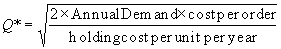Inventory Management Definition

Inventory -- stored resource (raw material, work-in-process, finished goods) that is used to satisfy present or future demand

Inventory management -- determine how much to order? When to order?

ABC Analysis -- classify inventory into 3 groups according to its annual dollar volume/usage

Annual dollar volume = annual demand x cost

An example:

 A Top 80% of total dollar volume B Next 15% C Next 5%
 Item# Annual Demand Cost Demand x Cost % of total cost Class 234 50 200 10000 10% B 170 10 200 2000 2% C 222 100 800 80000 80% A 410 50 100 5000 5% B 160 15 200 3000 3% C Total 100000

Exercise

Pg.541 Problem 13, 27

Purposes of inventory

1.  Smooth-out variations in operation performances

2.  Avoid stock out or shortage

3.  Safeguard against price changes and inflation

4.  Take advantage of quantity discounts

Inventory costs

1.  Holding or carrying costs: storage, insurance, investment, pilferage, etc.

Annual holding cost = average inventory level x holding cost per unit per year
= order quantity/2 x holding cost per unit per year

2.  Setup or ordering costs: cost involved in placing an order or setting up the equipment to make the product

Annual ordering cost = no. of orders placed in a year x cost per order
= annual demand/order quantity x cost per order

EOQ (Economic Order Quantity) Model

Assumptions

1.  Order arrives instantly

2.  No stockout

3.  Constant rate of demand

What is the order quantity such that the total cost is minimized?

1.  Total cost = holding cost + ordering cost

= (order quantity/2) x holding cost per unit per year + (annual demand/order quantity) x cost per order

2.  Optimal order quantity (Q*) is found when annual holding cost = ordering cost3.  Number of orders = Annual Demand/Q*

4.  Time between orders = No. of working days per year / number of orders

5.  Reorder point = daily demand x lead time + safety stock

Example:

Given:

Annual Demand = 60,000
Ordering cost = \$25 per order
Holding cost = \$3 per item per year
No. of working days per year = 240
Then, it can be computed:
Q* = 1000

Total cost = \$3000

Number of orders = 60000/1000 = 60

Time between orders = 240/60 = 4 days

Daily demand = 60000/240 = 250

If lead time = 3 days (lead time < time between orders)

Reorder point = (60000/240)x3=750
Reorder when inventory on hand = 750

If lead time = 5 days (lead time > time between orders)

Reorder point = 250x5 = 1250
Reorder when inventory on hand = 1250-Q*=1250-1000=250

In class exercise

Pg.540, Problems 10
Annual demand = 2000
Ordering cost = \$10
Holding cost = \$5

EOQ = sqrt(2*2000*10/5) = 89
Annual ordering cost = 2000/89*\$10 = \$223.6
Annual holding cost = 89/2*\$5 = \$223.6

Exercise

Pg. 539, Problem 1, 7a

Quantity Discount Model

1.  Total cost = holding + ordering + purchasing

2.  Holding cost is a % of the purchasing cost

Case 1
Annual Demand =100 per year

Ordering cost = 45 per order

Holding cost = 20% of cost of item

 Order quantity Cost per item 50 or less \$18 51 to 59 \$16 60 or more \$12à Should order 62 units

Case 2

Same as case 1 except:

 Order quantity Cost per item EOQ Remark 50 or less \$18 50 51 to 99 \$16 54 100 or more \$12 62 Infeasible

Need to compare:

Total cost (Q=54) and Total cost (Q=100)

Total cost (Q=54) = (100/54)x45 + (54/2)x(0.2x16) + 16x100 =1780.53

Total cost (Q=100) = (100/100)x45 + (100/2)x(0.2x12) + 12x100 = 1425

à Order 100 units

Case 3

Same as case 1 except:

 Order quantity Cost per item EOQ Remark 55 or less \$18 50 Feasible 56 to 99 \$16 54 Infeasible 100 or more \$12 62 Infeasible

Need to compare:

Total cost (Q=50), Total cost (Q=56) and Total cost (Q=100)

Total cost (Q=50) = (100/50)x45 + (50/2)x(0.2x18) + 18x100 = 1980

Total cost (Q=56) = (100/56)x45 + (56/2)x(0.2x16) + 16x100 =1781.16

Total cost (Q=100) = 1425

à Order 100 units

Pg.540, problem 7b

Exercise

Pg. 540, problems 12, 26